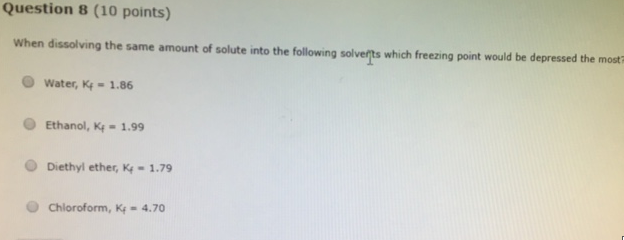# Problem: Question 8 (10 points) When dissolving the same amount of solute into the following solvents which freezing point would be depressed the most? (i) Water, Kf = 1.86 (ii) Ethanol, Kf =  1.99 (iii) Diethyl ether, Kf = 1.79 (iv) Chloroform, Kf = 4.70

###### FREE Expert Solution

Recall that ΔTf = Kf • m • i, which tells us that Kf is directly proportional to the depression. Therefore

100% (447 ratings)###### Problem Details

Question 8 (10 points)

When dissolving the same amount of solute into the following solvents which freezing point would be depressed the most?

(i) Water, Kf = 1.86

(ii) Ethanol, Kf =  1.99

(iii) Diethyl ether, Kf = 1.79

(iv) Chloroform, Kf = 4.70